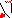# MINLPLib

### A Library of Mixed-Integer and Continuous Nonlinear Programming Instances

#### Instance: ex7_3_4

 Formatsⓘ ams gms mod nl osil pip Primal Boundsⓘ 10.00000000 p1 ( gdx sol ) (infeas: 0)8.46047227 p2 ( gdx sol ) (infeas: 8e-10)6.27463434 p3 ( gdx sol ) (infeas: 5e-13) Dual Boundsⓘ 6.27463433 (ANTIGONE)6.27463432 (BARON)6.27463434 (COUENNE)6.27463434 (LINDO)6.27463384 (SCIP) Referencesⓘ Floudas, C A, Pardalos, Panos M, Adjiman, C S, Esposito, W R, Gumus, Zeynep H, Harding, S T, Klepeis, John L, Meyer, Clifford A, and Schweiger, C A, Handbook of Test Problems in Local and Global Optimization, Kluwer Academic Publishers, 1999.Vicino, A, Tesi, A, and Milanese, M, Computation of Nonconservative Stability Perturbation Bounds for Systems with Nonlinearly Correlated Uncertainties, IEEE Transactions on Automatic Control, 35:7, 1990, 835-841. Sourceⓘ Test Problem ex7.3.4 of Chapter 7 of Floudas e.a. handbook Added to libraryⓘ 31 Jul 2001 Problem typeⓘ NLP #Variablesⓘ 12 #Binary Variablesⓘ 0 #Integer Variablesⓘ 0 #Nonlinear Variablesⓘ 9 #Nonlinear Binary Variablesⓘ 0 #Nonlinear Integer Variablesⓘ 0 Objective Senseⓘ min Objective typeⓘ linear Objective curvatureⓘ linear #Nonzeros in Objectiveⓘ 1 #Nonlinear Nonzeros in Objectiveⓘ 0 #Constraintsⓘ 17 #Linear Constraintsⓘ 10 #Quadratic Constraintsⓘ 1 #Polynomial Constraintsⓘ 6 #Signomial Constraintsⓘ 0 #General Nonlinear Constraintsⓘ 0 Operands in Gen. Nonlin. Functionsⓘ Constraints curvatureⓘ indefinite #Nonzeros in Jacobianⓘ 51 #Nonlinear Nonzeros in Jacobianⓘ 23 #Nonzeros in (Upper-Left) Hessian of Lagrangianⓘ 25 #Nonzeros in Diagonal of Hessian of Lagrangianⓘ 3 #Blocks in Hessian of Lagrangianⓘ 2 Minimal blocksize in Hessian of Lagrangianⓘ 4 Maximal blocksize in Hessian of Lagrangianⓘ 5 Average blocksize in Hessian of Lagrangianⓘ 4.5 #Semicontinuitiesⓘ 0 #Nonlinear Semicontinuitiesⓘ 0 #SOS type 1ⓘ 0 #SOS type 2ⓘ 0 Infeasibility of initial pointⓘ 10 Sparsity JacobianⓘSparsity Hessian of Lagrangianⓘ```\$offlisting
*
*  Equation counts
*      Total        E        G        L        N        X        C        B
*         18        8        0       10        0        0        0        0
*
*  Variable counts
*                   x        b        i      s1s      s2s       sc       si
*      Total     cont   binary  integer     sos1     sos2    scont     sint
*         13       13        0        0        0        0        0        0
*  FX      0
*
*  Nonzero counts
*      Total    const       NL      DLL
*         53       30       23        0
*
*  Solve m using NLP minimizing objvar;

Variables  x1,x2,x3,x4,x5,x6,x7,x8,x9,x10,x11,x12,objvar;

Positive Variables  x11;

Equations  e1,e2,e3,e4,e5,e6,e7,e8,e9,e10,e11,e12,e13,e14,e15,e16,e17,e18;

e1..  - x12 + objvar =E= 0;

e2.. POWER(x11,4)*x10 - sqr(x11)*x8 + x6 =E= 0;

e3.. sqr(x11)*x9 - x7 =E= 0;

e4..  - x1 - x12 =L= -10;

e5..    x1 - x12 =L= 10;

e6..    x2 - 0.1*x12 =L= 1;

e7..  - x2 - 0.1*x12 =L= -1;

e8..  - x3 - 0.1*x12 =L= -1;

e9..    x3 - 0.1*x12 =L= 1;

e10..  - x4 - 0.01*x12 =L= -0.2;

e11..    x4 - 0.01*x12 =L= 0.2;

e12..  - x5 - 0.005*x12 =L= -0.05;

e13..    x5 - 0.005*x12 =L= 0.05;

e14.. -54.387*x3*x2 + x6 =E= 0;

e15.. -0.2*(1364.67*x3*x2 - 147.15*x4*x3*x2) + 5.544*x5 + x7 =E= 0;

e16.. -3*(-9.81*sqr(x2)*x3 - 9.81*x3*x1*x2 - 4.312*sqr(x3)*x2 + 264.896*x3*x2
+ x4*x5 - 9.274*x5) + x8 =E= 0;

e17.. -(7*sqr(x3)*x4*x2 - 64.918*sqr(x3)*x2 + 380.067*x3*x2 + 3*x5*x2 + 3*x5*x1
) + x9 =E= 0;

e18.. -sqr(x3)*x2*(7*x1 + 4*x2) + x10 =E= 0;

* set non-default bounds
x11.up = 10;

Model m / all /;

m.limrow=0; m.limcol=0;
m.tolproj=0.0;

\$if NOT '%gams.u1%' == '' \$include '%gams.u1%'

\$if not set NLP \$set NLP NLP
Solve m using %NLP% minimizing objvar;

```

Last updated: 2019-02-14 Git hash: a71254dc